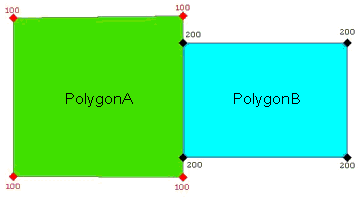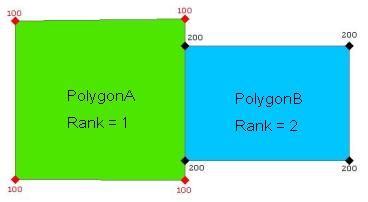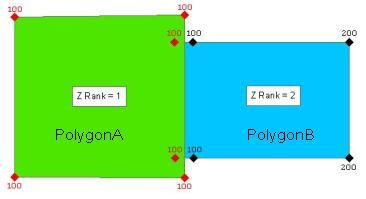English

# FAQ: How Zs Aware polygons are handled in Validate Topology at 8.3

## Question

How Zs Aware polygons are handled in Validate Topology at 8.3

INTRODUCTION

Point, line and polygon feature classes inside of the geodatabase can contain shape attributes. Shape attributes include elevation values (Z value), measure values (M value), and Point IDS. All of these attributes are stored on the vertex of each feature in a feature class that contains shape attributes.

Simple point, line and polygon feature classes with or without shape attributes can participate in a topology. Topology is used to measure and improve the quality and spatial accuracy of features in its participating feature classes. Topology uses a process called validation to measure and ensure correctness of features. Validation snaps geometry between features together, given a tolerance value.

This document describes how Z values are altered when validating a topology in ArcGIS 8.3.

`Note:The handling of Z values for ArcGIS 8.3, as described in this article, will change. Future versions of ArcGIS, such as 9.0 or 9.1 will describe a new implementation of this function.`

VERTEX ATTRIBUTES INTERPOLATION AND COLLISION

During the validation process two operations are used to determine the Zs of vertices; interpolation and collision.

INTERPOLATION

Interpolation is used to determine the vertex attributes of the new vertices when a segment is subdivided.

The interpolation for Z’s is :

Zi = FZ1 + (1-F)Z2

where Zi is the interpolated measure, Z1 and Z2 are the measures at the end points, and F is the ratio of the subdivision. Ranges are between 0.0 and 1.0, which means that at F = 0.5 the average is computed.

COLLISION

Different vertices with the same XY are represented by the same point. When two points are joined together because of this, they are said to 'collide'. In a case of collision there are two possibilities: the Z-ranks are equal or they are not.

If the ranks are not equal, the resulting point is assigned the Z-value and Z-rank of the point with the highest rank.

If the ranks are equal, a weighted Z average is assigned to the output points.

`Note:Vertices on polylines never collide because they live in different planes. For polygons, newly created vertices can collide because they are considered to be in the same plane (planar representation).`

EXAMPLE 1: POLYGONZs : EQUAL Z RANKS

For Zs (Equal Z ranks) aware polygons the attributes of the vertices created by cracking/clustering or the shared vertices are determined by a weighted linear interpolation followed by a weighted average of the coincident vertices at a specific location. Refer to the section above: Vertex attributes Interpolation and Collision.

ORIGINAL FEATURESIn this example the original features contain constant Zs values and have the same ranks. Polygon A has a constant Zs value of 100 for all vertices, and polygon B a value of 200.

AFTER VALIDATEDETAILED STEPS: Equal Zs Ranks

STEP 1 (interpolation): The point A2 is interpolated on the line segment A0-A3. The equation used is Zi = FZ1+ (1-F)Z2. Because Z1 and Z2 are both 100, the value of F is irrelevant, and Zi will be 100 as well.

STEP 2 (interpolation): Computation of the point A1, which is interpolated on the line segment A0-A2. This proceeds as under step 1, with the same output.

STEP 3 (collision): Computation of the weighted average for points A2 and B2. Because both points will have a weight of 1 (A2 interpolated, B2 initially assigned), the output Z will be the average of 100 and 200, or 150.

STEP 4 (collision): Computation of the weighted average for points A1 and B1. This is the same as step 3.

EXAMPLE 2: POLYGONZs UNEQUAL Zs RANKS

When working with Zs aware data validate is introducing a new concept: the Zs rank. If there are z-aware feature classes in the topology, rank them so that the z-values of vertices collected with higher accuracy are not changed when snapping with the z-values of features of lower rank. A rank of 1 is the highest rank. Refer to the section above: Vertex attributes Interpolation and Collision.

ORIGINAL FEATURESIn this example the original features contain constant Zs values. Polygon A has a constant Zs value of 100 for all vertices, and polygon B has a value of 200. The polygon A has a rank of 1 and the polygon B has a rank of 2.

AFTER VALIDATEIn this example after validate the features attributes are modified. The polygon A has a higher rank (rank of 1) than the polygon B (rank of 2). The interpolation steps are the same as in the previous example. The difference is in the collision step, the resulting Z values will be those of the points of polygon A, because it has the higher rank.